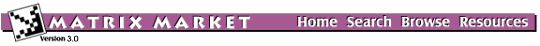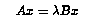## BFWAVE: Bounded Finline Dielectric Waveguide

### from the NEP Collection

Set BFWAVE
Source: B. Schultz and S. Gedney, University of Kentucky
Discipline: Electrical engineering

Millimeter wave technology has been applied in radar, communication, radiometry and instruments. The finline waveguide is an example of a bounded waveguide which operates extremely well in the millimeter wave spectrum. The generalized eigenvalue problemstudied in [Schultz] arises in the finite element analysis of Maxwell's equation (see [Fernandez and Lu] and [Jin]) for finding the propagating modes and magnetic field profiles of a rectangular waveguide filled with dielectric and PEC structures. The eigenvalues and corresponding eigenvectors of interest are the ones with positive real parts, which correspond to the propagation modes of a waveguide. The matrix A is non-symmetric and B is symmetric positive definite. Though real data is collected here, in applications, complex matrices may be involved.

Matrices in this set come in pairs, corresponding to the matrices in the generalized eigenproblem Ax = (lamba)Bx. The matrices are named BFWnnnnA and BFWnnnnB, respectively.

## Matrices in this set:

The Matrix Market is a service of the Mathematical and Computational Sciences Division / Information Technology Laboratory / National Institute of Standards and Technology.

[ Home ] [ Search ] [ Browse ] [ Resources ]